How to read this lecture...

Code should execute sequentially if run in a Jupyter notebook

• See the set up page to install Jupyter, Julia (1.0+) and all necessary libraries
• Please direct feedback to contact@quantecon.org or the discourse forum
• For some notebooks, enable content with "Trust" on the command tab of Jupyter lab
• If using QuantEcon lectures for the first time on a computer, execute ] add InstantiateFromURL inside of a notebook or the REPL

# Multiplicative Functionals¶

## Contents¶

Co-authored with Chase Coleman and Balint Szoke

## Overview¶

This lecture is a sequel to the lecture on additive functionals

That lecture

1. defined a special class of additive functionals driven by a first-order vector VAR
2. by taking the exponential of that additive functional, created an associated multiplicative functional

This lecture uses this special class to create and analyze two examples

• A log likelihood process, an object at the foundation of both frequentist and Bayesian approaches to statistical inference
• A version of Robert E. Lucas’s [Luc03] and Thomas Tallarini’s [Tal00] approaches to measuring the benefits of moderating aggregate fluctuations

## A Log-Likelihood Process¶

Consider a vector of additive functionals $\{y_t\}_{t=0}^\infty$ described by

\begin{aligned} x_{t+1} & = A x_t + B z_{t+1} \\ y_{t+1} - y_t & = D x_{t} + F z_{t+1}, \end{aligned}

where $A$ is a stable matrix, $\{z_{t+1}\}_{t=0}^\infty$ is an i.i.d. sequence of ${\cal N}(0,I)$ random vectors, $F$ is nonsingular, and $x_0$ and $y_0$ are vectors of known numbers

Evidently,

$$x_{t+1} = \left(A - B F^{-1}D \right)x_t + B F^{-1} \left(y_{t+1} - y_t \right),$$

so that $x_{t+1}$ can be constructed from observations on $\{y_{s}\}_{s=0}^{t+1}$ and $x_0$

The distribution of $y_{t+1} - y_t$ conditional on $x_t$ is normal with mean $Dx_t$ and nonsingular covariance matrix $FF'$

Let $\theta$ denote the vector of free parameters of the model

These parameters pin down the elements of $A, B, D, F$

The log likelihood function of $\{y_s\}_{s=1}^t$ is

\begin{aligned} \log L_{t}(\theta) = & - {\frac 1 2} \sum_{j=1}^{t} (y_{j} - y_{j-1} - D x_{j-1})'(FF')^{-1}(y_{j} - y_{j-1} - D x_{j-1}) \\ & - {\frac t 2} \log \det (FF') - {\frac {k t} 2} \log( 2 \pi) \end{aligned}

Let’s consider the case of a scalar process in which $A, B, D, F$ are scalars and $z_{t+1}$ is a scalar stochastic process

We let $\theta_o$ denote the “true” values of $\theta$, meaning the values that generate the data

For the purposes of this exercise, set $\theta_o = (A, B, D, F) = (0.8, 1, 0.5, 0.2)$

Set $x_0 = y_0 = 0$

### Simulating sample paths¶

Let’s write a program to simulate sample paths of $\{ x_t, y_{t} \}_{t=0}^{\infty}$

We’ll do this by formulating the additive functional as a linear state space model and putting the LSS struct to work

### Setup¶

In :
using InstantiateFromURL
github_project("QuantEcon/quantecon-notebooks-julia", version = "0.3.0")

In :
using LinearAlgebra, Statistics
using Distributions, Parameters, Plots, QuantEcon
import Distributions: loglikelihood
gr(fmt = :png);

In :
AMF_LSS_VAR = @with_kw (A, B, D, F = 0.0, ν = 0.0, lss = construct_ss(A, B, D, F, ν))

function construct_ss(A, B, D, F, ν)
H, g = additive_decomp(A, B, D, F)

# Build A matrix for LSS
# Order of states is: [1, t, xt, yt, mt]
A1 = [1 0 0 0 0]       # Transition for 1
A2 = [1 1 0 0 0]       # Transition for t
A3 = [0 0 A 0 0]       # Transition for x_{t+1}
A4 = [ν 0 D 1 0]       # Transition for y_{t+1}
A5 = [0 0 0 0 1]       # Transition for m_{t+1}
Abar = vcat(A1, A2, A3, A4, A5)

# Build B matrix for LSS
Bbar = [0, 0, B, F, H]

# Build G matrix for LSS
# Order of observation is: [xt, yt, mt, st, tt]
G1 = [0 0 1 0 0]               # Selector for x_{t}
G2 = [0 0 0 1 0]               # Selector for y_{t}
G3 = [0 0 0 0 1]               # Selector for martingale
G4 = [0 0 -g 0 0]              # Selector for stationary
G5 = [0 ν 0 0 0]               # Selector for trend
Gbar = vcat(G1, G2, G3, G4, G5)

# Build LSS struct
x0 = [0, 0, 0, 0, 0]
S0 = zeros(5, 5)
return LSS(Abar, Bbar, Gbar, mu_0 = x0, Sigma_0 = S0)
end

function additive_decomp(A, B, D, F)
A_res = 1 / (1 - A)
g = D * A_res
H = F + D * A_res * B

return H, g
end

function multiplicative_decomp(A, B, D, F, ν)
H, g = additive_decomp(A, B, D, F)
ν_tilde = ν + 0.5 * H^2

return ν_tilde, H, g
end

function loglikelihood_path(amf, x, y)
@unpack A, B, D, F = amf
T = length(y)
FF = F^2
FFinv = inv(FF)
temp = y[2:end] - y[1:end-1] - D*x[1:end-1]
obs =  temp .* FFinv .* temp
obssum = cumsum(obs)
scalar = (log(FF) + log(2pi)) * (1:T-1)
return -0.5 * (obssum + scalar)
end

function loglikelihood(amf, x, y)
llh = loglikelihood_path(amf, x, y)
return llh[end]
end

Out:
loglikelihood (generic function with 4 methods)

The heavy lifting is done inside the AMF_LSS_VAR struct

The following code adds some simple functions that make it straightforward to generate sample paths from an instance of AMF_LSS_VAR

In :
function simulate_xy(amf, T)
foo, bar = simulate(amf.lss, T)
x = bar[1, :]
y = bar[2, :]
return x, y
end

function simulate_paths(amf, T = 150, I = 5000)
# Allocate space
storeX = zeros(I, T)
storeY = zeros(I, T)

for i in 1:I
# Do specific simulation
x, y = simulate_xy(amf, T)

# Fill in our storage matrices
storeX[i, :] = x
storeY[i, :] = y
end

return storeX, storeY
end

function population_means(amf, T = 150)
# Allocate Space
xmean = zeros(T)
ymean = zeros(T)

# Pull out moment generator
moment_generator = moment_sequence(amf.lss)
for (tt, x) = enumerate(moment_generator)
ymeans = x
xmean[tt] = ymeans
ymean[tt] = ymeans
if tt == T
break
end
end
return xmean, ymean
end

Out:
population_means (generic function with 2 methods)

Now that we have these functions in our took kit, let’s apply them to run some simulations

In particular, let’s use our program to generate $I = 5000$ sample paths of length $T = 150$, labeled $\{ x_{t}^i, y_{t}^i \}_{t=0}^\infty$ for $i = 1, ..., I$

Then we compute averages of $\frac{1}{I} \sum_i x_t^i$ and $\frac{1}{I} \sum_i y_t^i$ across the sample paths and compare them with the population means of $x_t$ and $y_t$

Here goes

In :
F = 0.2
amf = AMF_LSS_VAR(A = 0.8, B = 1.0, D = 0.5, F = F)

T = 150
I = 5000

# Simulate and compute sample means
Xit, Yit = simulate_paths(amf, T, I)
Xmean_t = mean(Xit, dims = 1)
Ymean_t = mean(Yit, dims = 1)

# Compute population means
Xmean_pop, Ymean_pop = population_means(amf, T)

# Plot sample means vs population means
plt_1 = plot(Xmean_t', color = :blue, label = "1/I sum_i x_t^i")
plot!(plt_1, Xmean_pop, color = :black, label = "E x_t")
plot!(plt_1, title = "x_t", xlim = (0, T), legend = :bottomleft)

plt_2 = plot(Ymean_t', color = :blue, label = "1/I sum_i x_t^i")
plot!(plt_2, Ymean_pop, color = :black, label = "E y_t")
plot!(plt_2, title = "y_t", xlim = (0, T), legend = :bottomleft)

plot(plt_1, plt_2, layout = (2, 1), size = (800,500))

Out: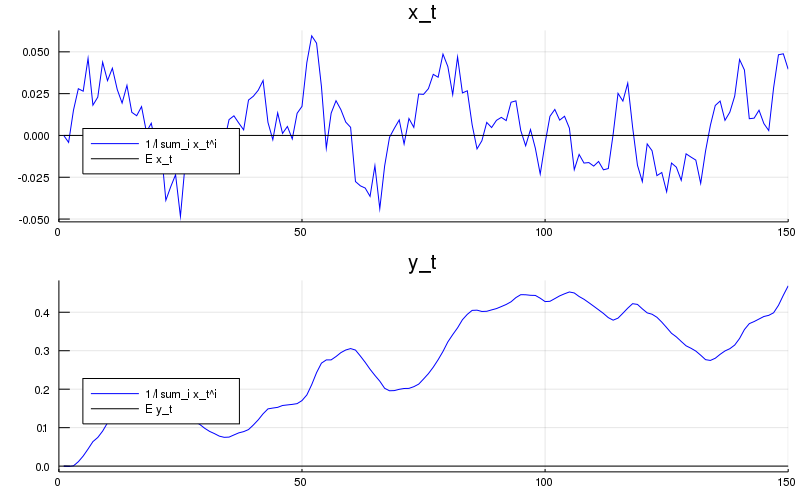### Simulating log-likelihoods¶

Our next aim is to write a program to simulate $\{\log L_t \mid \theta_o\}_{t=1}^T$

We want as inputs to this program the same sample paths $\{x_t^i, y_t^i\}_{t=0}^T$ that we have already computed

We now want to simulate $I = 5000$ paths of $\{\log L_t^i \mid \theta_o\}_{t=1}^T$

• For each path, we compute $\log L_T^i / T$
• We also compute $\frac{1}{I} \sum_{i=1}^I \log L_T^i / T$

Then we to compare these objects

Below we plot the histogram of $\log L_T^i / T$ for realizations $i = 1, \ldots, 5000$

In :
function simulate_likelihood(amf, Xit, Yit)
# Get size
I, T = size(Xit)

# Allocate space
LLit = zeros(I, T-1)

for i in 1:I
LLit[i, :] = loglikelihood_path(amf, Xit[i, :], Yit[i, :])
end

return LLit
end

# Get likelihood from each path x^{i}, Y^{i}
LLit = simulate_likelihood(amf, Xit, Yit)

LLT = 1 / T * LLit[:, end]
LLmean_t = mean(LLT)

plot(seriestype = :histogram, LLT, label = "")
plot!(title = "Distribution of (I/T)log(L_T)|theta_0")
vline!([LLmean_t], linestyle = :dash, color = :black, lw = 2, alpha = 0.6, label = "")

Out: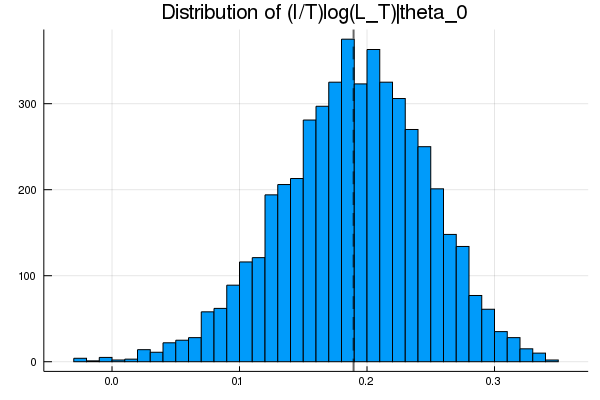Notice that the log likelihood is almost always nonnegative, implying that $L_t$ is typically bigger than 1

Recall that the likelihood function is a pdf (probability density function) and not a probability measure, so it can take values larger than 1

In the current case, the conditional variance of $\Delta y_{t+1}$, which equals $FF^T=0.04$, is so small that the maximum value of the pdf is 2 (see the figure below)

This implies that approximately $75\%$ of the time (a bit more than one sigma deviation), we should expect the increment of the log likelihood to be nonnegative

Let’s see this in a simulation

In :
normdist = Normal(0, F)
mult = 1.175
println("The pdf at +/- $mult sigma takes the value:$(pdf(normdist,mult*F))")
println("Probability of dL being larger than 1 is approx: "*
"$(cdf(normdist,mult*F)-cdf(normdist,-mult*F))") # Compare this to the sample analogue: L_increment = LLit[:,2:end] - LLit[:,1:end-1] r,c = size(L_increment) frac_nonegative = sum(L_increment.>=0)/(c*r) print("Fraction of dlogL being nonnegative in the sample is:$(frac_nonegative)")

The pdf at +/- 1.175 sigma takes the value: 1.0001868966924388
Probability of dL being larger than 1 is approx: 0.7600052842019751
Fraction of dlogL being nonnegative in the sample is: 0.7610513513513514

Let’s also plot the conditional pdf of $\Delta y_{t+1}$

In :
xgrid = range(-1,  1, length = 100)
println("The pdf at +/- one sigma takes the value: $(pdf(normdist, F)) ") plot(xgrid, pdf.(normdist, xgrid), label = "") plot!(title = "Conditional pdf f(Delta y_(t+1) | x_t)")  The pdf at +/- one sigma takes the value: 1.2098536225957168  Out: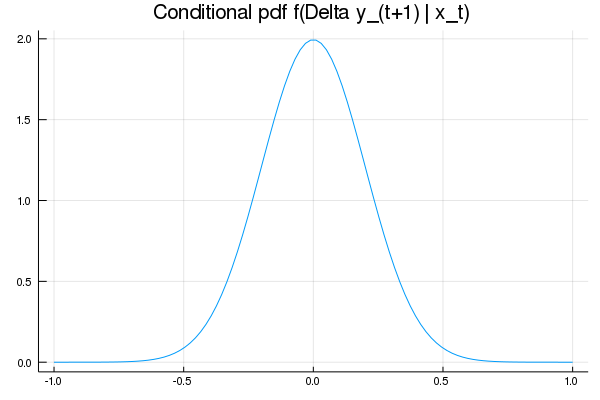### An alternative parameter vector¶ Now consider alternative parameter vector$ \theta_1 = [A, B, D, F] = [0.9, 1.0, 0.55, 0.25] $We want to compute$ \{\log L_t \mid \theta_1\}_{t=1}^T $The$ x_t, y_t $inputs to this program should be exactly the same sample paths$ \{x_t^i, y_t^i\}_{t=0}^T $that we we computed above This is because we want to generate data under the$ \theta_o $probability model but evaluate the likelihood under the$ \theta_1 $model So our task is to use our program to simulate$ I = 5000 $paths of$ \{\log L_t^i \mid \theta_1\}_{t=1}^T $• For each path, compute$ \frac{1}{T} \log L_T^i $• Then compute$ \frac{1}{I}\sum_{i=1}^I \frac{1}{T} \log L_T^i $We want to compare these objects with each other and with the analogous objects that we computed above Then we want to interpret outcomes A function that we constructed can handle these tasks The only innovation is that we must create an alternative model to feed in We will creatively call the new model amf2 We make three graphs • the first sets the stage by repeating an earlier graph • the second contains two histograms of values of log likelihoods of the two models over the period$ T $• the third compares likelihoods under the true and alternative models Here’s the code In : # Create the second (wrong) alternative model amf2 = AMF_LSS_VAR(A = 0.9, B = 1.0, D = 0.55, F = 0.25) # parameters for θ_1 closer to θ_0 # Get likelihood from each path x^{i}, y^{i} LLit2 = simulate_likelihood(amf2, Xit, Yit) LLT2 = 1/(T-1) * LLit2[:, end] LLmean_t2 = mean(LLT2) plot(seriestype = :histogram, LLT2, label = "") vline!([LLmean_t2], color = :black, lw = 2, linestyle = :dash, alpha = 0.6, label = "") plot!(title = "Distribution of (1/T)log(L_T) | theta_1)")  Out: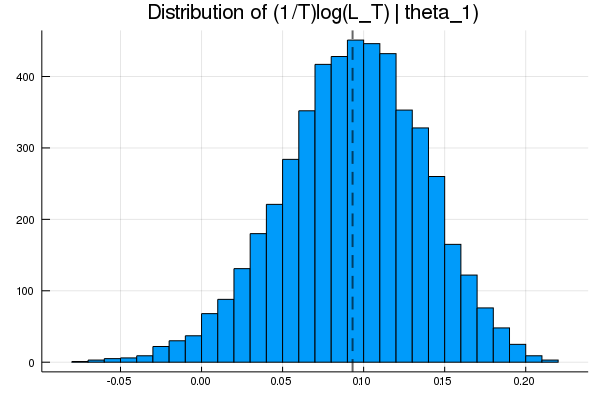Let’s see a histogram of the log-likelihoods under the true and the alternative model (same sample paths) In : plot(seriestype = :histogram, LLT, bin = 50, alpha = 0.5, label = "True", normed = true) plot!(seriestype = :histogram, LLT2, bin = 50, alpha = 0.5, label = "Alternative", normed = true) vline!([mean(LLT)], color = :black, lw = 2, linestyle = :dash, label = "") vline!([mean(LLT2)], color = :black, lw = 2, linestyle = :dash, label = "")  Out: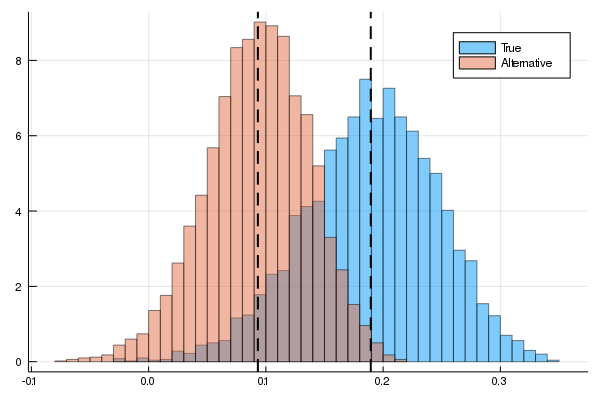Now we’ll plot the histogram of the difference in log likelihood ratio In : LLT_diff = LLT - LLT2 plot(seriestype = :histogram, LLT_diff, bin = 50, label = "") plot!(title = "(1/T)[log(L_T^i | theta_0) - log(L_T^i |theta_1)]")  Out: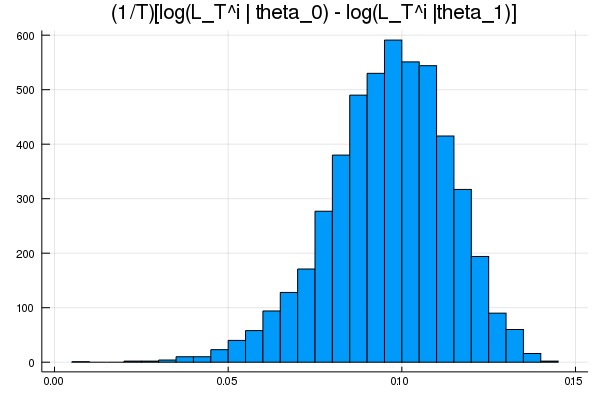### Interpretation¶ These histograms of log likelihood ratios illustrate important features of likelihood ratio tests as tools for discriminating between statistical models • The loglikeklihood is higher on average under the true model – obviously a very useful property • Nevertheless, for a positive fraction of realizations, the log likelihood is higher for the incorrect than for the true model • in these instances, a likelihood ratio test mistakenly selects the wrong model • These mechanics underlie the statistical theory of mistake probabilities associated with model selection tests based on likelihood ratio (In a subsequent lecture, we’ll use some of the code prepared in this lecture to illustrate mistake probabilities) ## Benefits from Reduced Aggregate Fluctuations¶ Now let’s turn to a new example of multiplicative functionals This example illustrates ideas in the literatures on • long-run risk in the consumption based asset pricing literature (e.g., [BY04], [HHL08], [Han07]) • benefits of eliminating aggregate fluctuations in representative agent macro models (e.g., [Tal00], [Luc03]) Let$ c_t $be consumption at date$ t \geq 0 $Suppose that$ \{\log c_t \}_{t=0}^\infty $is an additive functional described by $$\log c_{t+1} - \log c_t = \nu + D \cdot x_t + F \cdot z_{t+1}$$ where $$x_{t+1} = A x_t + B z_{t+1}$$ Here$ \{z_{t+1}\}_{t=0}^\infty $is an i.i.d. sequence of$ {\cal N}(0,I) $random vectors A representative household ranks consumption processes$ \{c_t\}_{t=0}^\infty $with a utility functional$ \{V_t\}_{t=0}^\infty $that satisfies $$\log V_t - \log c_t = U \cdot x_t + {\sf u} \tag{1}$$ where $$U = \exp(-\delta) \left[ I - \exp(-\delta) A' \right]^{-1} D$$ and $${\sf u} = {\frac {\exp( -\delta)}{ 1 - \exp(-\delta)}} {\nu} + \frac{(1 - \gamma)}{2} {\frac {\exp(-\delta)}{1 - \exp(-\delta)}} \biggl| D' \left[ I - \exp(-\delta) A \right]^{-1}B + F \biggl|^2,$$ Here$ \gamma \geq 1 $is a risk-aversion coefficient and$ \delta > 0 $is a rate of time preference ### Consumption as a multiplicative process¶ We begin by showing that consumption is a multiplicative functional with representation $$\frac{c_t}{c_0} = \exp(\tilde{\nu}t ) \left( \frac{\tilde{M}_t}{\tilde{M}_0} \right) \left( \frac{\tilde{e}(x_0)}{\tilde{e}(x_t)} \right) \tag{2}$$ where$ \left( \frac{\tilde{M}_t}{\tilde{M}_0} \right) $is a likelihood ratio process and$ \tilde M_0 = 1 $At this point, as an exercise, we ask the reader please to verify the follow formulas for$ \tilde{\nu} $and$ \tilde{e}(x_t) $as functions of$ A, B, D, F $: $$\tilde \nu = \nu + \frac{H \cdot H}{2}$$ and $$\tilde e(x) = \exp[g(x)] = \exp \bigl[ D' (I - A)^{-1} x \bigr]$$ ### Simulating a likelihood ratio process again¶ Next, we want a program to simulate the likelihood ratio process$ \{ \tilde{M}_t \}_{t=0}^\infty $In particular, we want to simulate 5000 sample paths of length$ T=1000 $for the case in which$ x $is a scalar and$ [A, B, D, F] = [0.8, 0.001, 1.0, 0.01] $and$ \nu = 0.005 $After accomplishing this, we want to display a histogram of$ \tilde{M}_T^i $for$ T=1000 $Here is code that accomplishes these tasks In : function simulate_martingale_components(amf, T = 1_000, I = 5_000) # Get the multiplicative decomposition @unpack A, B, D, F, ν, lss = amf ν, H, g = multiplicative_decomp(A, B, D, F, ν) # Allocate space add_mart_comp = zeros(I, T) # Simulate and pull out additive martingale component for i in 1:I foo, bar = simulate(lss, T) # Martingale component is third component add_mart_comp[i, :] = bar[3, :] end mul_mart_comp = exp.(add_mart_comp' .- (0:T-1) * H^2 / 2)' return add_mart_comp, mul_mart_comp end # Build model amf_2 = AMF_LSS_VAR(A = 0.8, B = 0.001, D = 1.0, F = 0.01, ν = 0.005) amc, mmc = simulate_martingale_components(amf_2, 1_000, 5_000) amcT = amc[:, end] mmcT = mmc[:, end] println("The (min, mean, max) of additive Martingale component in period T is") println("\t ($(minimum(amcT)), $(mean(amcT)),$(maximum(amcT)))")

println("The (min, mean, max) of multiplicative Martingale component in period T is")
println("\t ($(minimum(mmcT)),$(mean(mmcT)), $(maximum(mmcT)))")  The (min, mean, max) of additive Martingale component in period T is (-1.613317955324071, 0.006982488586911062, 1.636135037412871) The (min, mean, max) of multiplicative Martingale component in period T is (0.17804740296993868, 1.003834483098736, 4.5893919265142165)  #### Comments¶ • The preceding min, mean, and max of the cross-section of the date$ T $realizations of the multiplicative martingale component of$ c_t $indicate that the sample mean is close to its population mean of 1 • This outcome prevails for all values of the horizon$ T $• The cross-section distribution of the multiplicative martingale component of$ c $at date$ T $approximates a log normal distribution well • The histogram of the additive martingale component of$ \log c_t $at date$ T $approximates a normal distribution well Here’s a histogram of the additive martingale component In : plot(seriestype = :histogram, amcT, bin = 25, normed = true, label = "") plot!(title = "Histogram of Additive Martingale Component")  Out: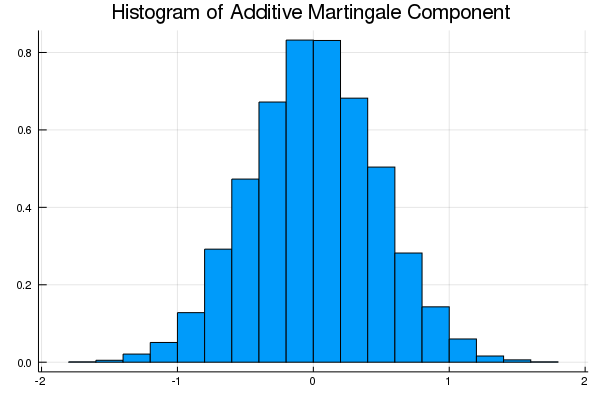Here’s a histogram of the multiplicative martingale component In : plot(seriestype = :histogram, mmcT, bin = 25, normed = true, label = "") plot!(title = "Histogram of Multiplicative Martingale Component")  Out: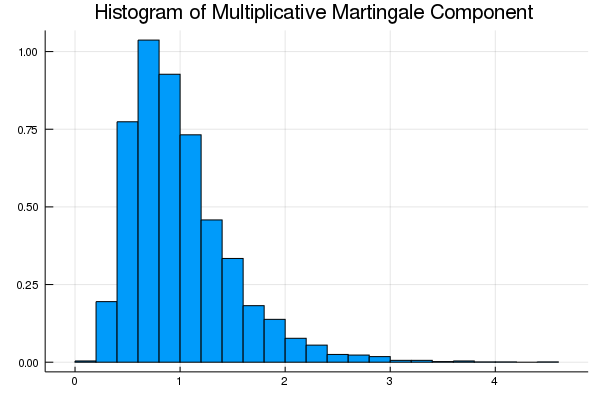### Representing the likelihood ratio process¶ The likelihood ratio process$ \{\widetilde M_t\}_{t=0}^\infty $can be represented as $$\widetilde M_t = \exp \biggl( \sum_{j=1}^t \biggl(H \cdot z_j -\frac{ H \cdot H }{2} \biggr) \biggr), \quad \widetilde M_0 =1 ,$$ where$ H = [F + B'(I-A')^{-1} D] $It follows that$ \log {\widetilde M}_t \sim {\mathcal N} ( -\frac{t H \cdot H}{2}, t H \cdot H ) $and that consequently$ {\widetilde M}_t $is log normal Let’s plot the probability density functions for$ \log {\widetilde M}_t $for$ t=100, 500, 1000, 10000, 100000 $Then let’s use the plots to investigate how these densities evolve through time We will plot the densities of$ \log {\widetilde M}_t $for different values of$ t $Here is some code that tackles these tasks In : function Mtilde_t_density(amf, t; xmin = 1e-8, xmax = 5.0, npts = 5000) # Pull out the multiplicative decomposition νtilde, H, g = multiplicative_decomp(amf.A, amf.B, amf.D, amf.F, amf.ν) H2 = H*H # The distribution mdist = LogNormal(-t * H2 / 2, sqrt(t * H2)) x = range(xmin, xmax, length = npts) p = pdf.(mdist, x) return x, p end function logMtilde_t_density(amf, t; xmin = -15.0, xmax = 15.0, npts = 5000) # Pull out the multiplicative decomposition @unpack A, B, D, F, ν = amf νtilde, H, g = multiplicative_decomp(A, B, D, F, ν) H2 = H * H # The distribution lmdist = Normal(-t * H2 / 2, sqrt(t * H2)) x = range(xmin, xmax, length = npts) p = pdf.(lmdist, x) return x, p end times_to_plot = [10, 100, 500, 1000, 2500, 5000] dens_to_plot = [Mtilde_t_density(amf_2, t, xmin=1e-8, xmax=6.0) for t in times_to_plot] ldens_to_plot = [logMtilde_t_density(amf_2, t, xmin=-10.0, xmax=10.0) for t in times_to_plot] # plot_title = "Densities of M_t^tilda" is required, however, plot_title is not yet # supported in Plots plots = plot(layout = (3,2), size = (600,800)) for (it, dens_t) in enumerate(dens_to_plot) x, pdf = dens_t plot!(plots[it], title = "Density for time (time_to_plot[it])") plot!(plots[it], pdf, fillrange = [, pdf], label = "") end plot(plots)  Out: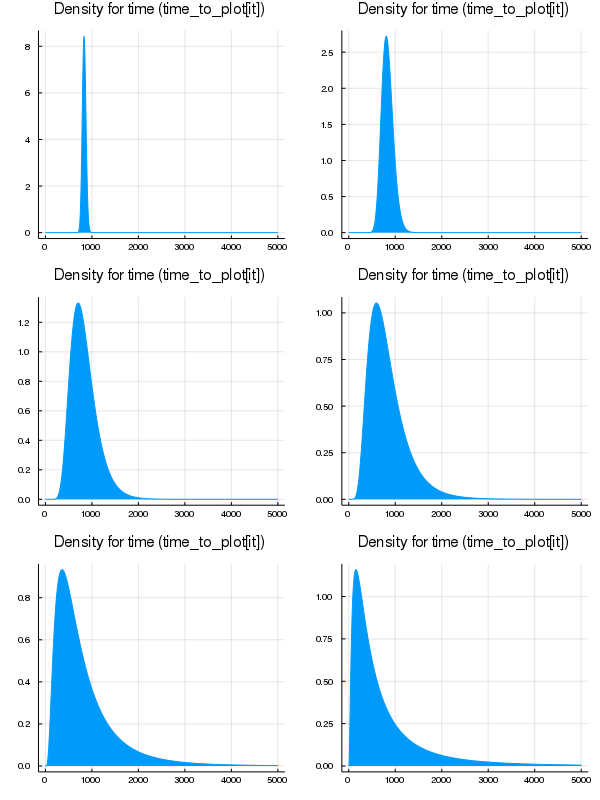These probability density functions illustrate a peculiar property of log likelihood ratio processes: • With respect to the true model probabilities, they have mathematical expectations equal to$ 1 $for all$ t \geq 0 $• They almost surely converge to zero ### Welfare benefits of reduced random aggregate fluctuations¶ Suppose in the tradition of a strand of macroeconomics (for example Tallarini [Tal00], [Luc03]) we want to estimate the welfare benefits from removing random fluctuations around trend growth We shall compute how much initial consumption$ c_0 $a representative consumer who ranks consumption streams according to (1) would be willing to sacrifice to enjoy the consumption stream $$\frac{c_t}{c_0} = \exp (\tilde{\nu} t)$$ rather than the stream described by equation (2) We want to compute the implied percentage reduction in$ c_0 $that the representative consumer would accept To accomplish this, we write a function that computes the coefficients$ U $and$ u $for the original values of$ A, B, D, F, \nu $, but also for the case that$ A, B, D, F = [0, 0, 0, 0] $and$ \nu = \tilde{\nu} Here’s our code In : function Uu(amf, δ, γ) @unpack A, B, D, F, ν = amf ν_tilde, H, g = multiplicative_decomp(A, B, D, F, ν) resolv = 1 / (1 - exp(-δ) * A) vect = F + D * resolv * B U_risky = exp(-δ) * resolv * D u_risky = exp(-δ) / (1 - exp(-δ)) * (ν + 0.5 * (1 - γ) * (vect^2)) U_det = 0 u_det = exp(-δ) / (1 - exp(-δ)) * ν_tilde return U_risky, u_risky, U_det, u_det end # Set remaining parameters δ = 0.02 γ = 2.0 # Get coeffs U_r, u_r, U_d, u_d = Uu(amf_2, δ, γ)  Out: (4.54129843114712, 0.24220854072375247, 0, 0.25307727077652764) The values of the two processes are \begin{aligned} \log V^r_0 &= \log c^r_0 + U^r x_0 + u^r \\ \log V^d_0 &= \log c^d_0 + U^d x_0 + u^d \end{aligned} We look for the ratio \frac{c^r_0-c^d_0}{c^r_0} $that makes$ \log V^r_0 - \log V^d_0 = 0 \begin{aligned} \underbrace{ \log V^r_0 - \log V^d_0}_{=0} + \log c^d_0 - \log c^r_0 &= (U^r-U^d) x_0 + u^r - u^d \\ \frac{c^d_0}{ c^r_0} &= \exp\left((U^r-U^d) x_0 + u^r - u^d\right) \end{aligned} Hence, the implied percentage reduction in c_0 \$ that the representative consumer would accept is given by

$$\frac{c^r_0-c^d_0}{c^r_0} = 1 - \exp\left((U^r-U^d) x_0 + u^r - u^d\right)$$

Let’s compute this

In :
x0 = 0.0  # initial conditions
logVC_r = U_r * x0 + u_r
logVC_d = U_d * x0 + u_d

perc_reduct = 100 * (1 - exp(logVC_r - logVC_d))
perc_reduct

Out:
1.0809878812017448

We find that the consumer would be willing to take a percentage reduction of initial consumption equal to around 1.081

• Share page